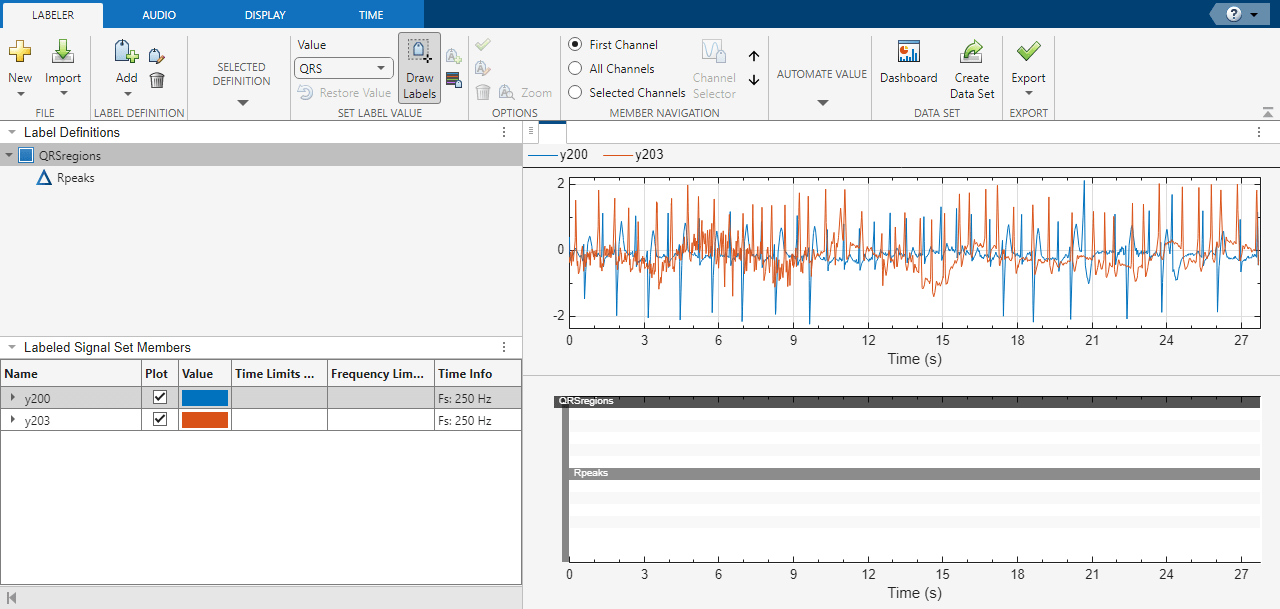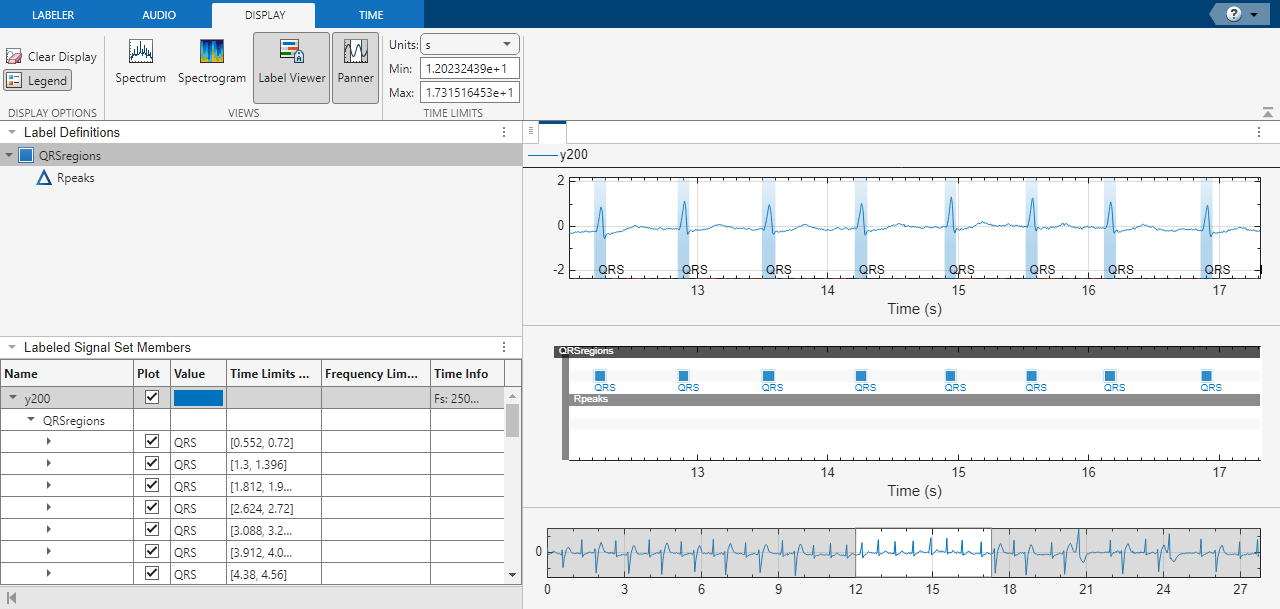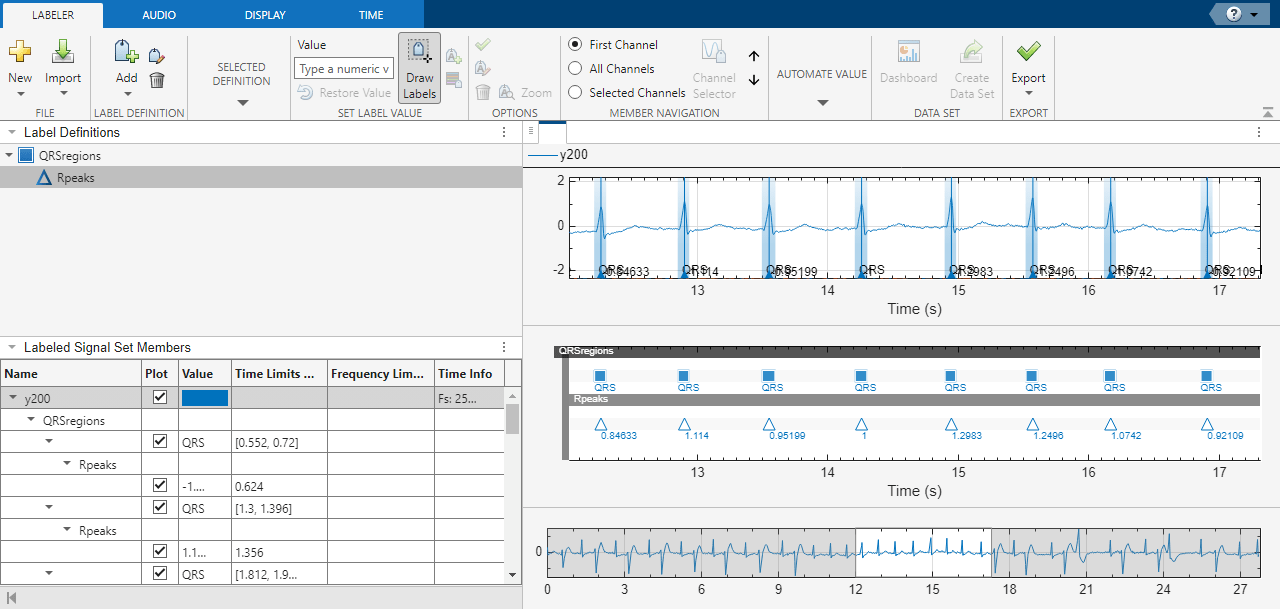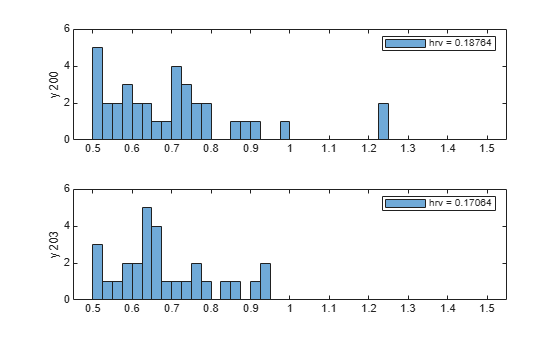# Label QRS Complexes and R Peaks of ECG Signals Using Deep Network

This example shows how to use custom autolabeling functions in Signal Labeler to label QRS complexes and R peaks of electrocardiogram (ECG) signals. One custom function uses a previously trained recurrent deep learning network to identify and locate the QRS complexes. Another custom function uses a simple peak finder to locate the R peaks. In this example, the network labels the QRS complexes of two signals that are completely independent of the network training and testing process.

The QRS complex, which consists of three deflections in the ECG waveform, reflects the depolarization of the right and left ventricles of the heart. The QRS is also the highest-amplitude segment of the human heartbeat. Study of the QRS complex can help assess the overall health of a person's heart and the presence of abnormalities . In particular, by locating R peaks within the QRS complexes and looking at the time intervals between consecutive peaks, a diagnostician can compute the heart-rate variability of a patient and detect cardiac arrhythmia.

The deep learning network in this example was introduced in Waveform Segmentation Using Deep Learning, where it was trained using ECG signals from the publicly available QT Database  . The data consists of roughly 15 minutes of ECG recordings from a total of 105 patients, sampled at 250 Hz. To obtain each recording, the examiners placed two electrodes on different locations on a patient's chest, which resulted in a two-channel signal. The database provides signal region labels generated by an automated expert system . The added labels make it possible to use the data to train a deep learning network.

### Load, Resample, and Import Data into Signal Labeler

The signals labeled in this example are from the MIT-BIH Arrhythmia Database . Each signal in the database was irregularly sampled at a mean rate of 360 Hz and was annotated by two cardiologists, allowing for verification of the results.

Load two MIT database signals, corresponding to records 200 and 203. Resample the signals to a uniform grid with a sample time of 1/250 second, which corresponds to the nominal sample rate of the QT Database data.

```load mit200 y200 = resample(ecgsig,tm,250); load mit203 y203 = resample(ecgsig,tm,250);```

Open Signal Labeler. On the Labeler tab, click Import ▼ and select Members From Workspace. In the dialog box, select the signals `y200` and `y203`. Add time information: Select `Time` from the drop-down list and specify a sample rate of 250 Hz. Click Import and close the dialog box. The signals appear in the Labeled Signal Set browser. Plot the signals by selecting the check boxes next to their names.

### Define Labels

Define labels to attach to the signals.

1. Define a categorical region-of-interest (ROI) label for the QRS complexes. Click Add Definition on the Labeler tab. Specify the Label Name as `QRSregions`, select a Label Type of `ROI`, enter the Data Type as `categorical`, and add two Categories, `QRS` and `n/a`, each on its own line.

2. Define a sublabel of `QRSregions` as a numerical point label for the R peaks. Click QRSregions in the Label Definitions browser to select it. Click Add Definition ▼ and select `Add sublabel definition`. Specify the Label Name as `Rpeaks`, select a LabelType of `Point`, and enter the Data Type as `numeric`.### Create Custom Autolabeling Functions

Create two Custom Labeling Functions, one to locate and label the QRS complexes and another to locate and label the R peak within each QRS complex. (Code for the `findQRS`, `computeFSST`, `p2qrs`, and `findRpeaks` functions appears later in the example.) To create each function, in the Labeler tab, click Automate Value ▼ and select Add Custom Function. Signal Labeler shows a dialog box asking for the name, description, and label type of the function.

1. For the function that locates the QRS complexes, enter `findQRS` in the Name field and select `ROI` as the Label Type. You can leave the Description field empty or you can enter your own description.

2. For the function that locates the R peaks, enter `findRpeaks` in the Name field and select `Point` as the Label Type. You can leave the Description field empty or you can enter your own description.

If you already have written the functions, and the functions are in the current folder or in the MATLAB® path, Signal Labeler adds the functions to the gallery. If you have not written the functions, Signal Labeler opens blank templates in the Editor for you to type or paste the code. Save the files. Once you save the files, the functions appear in the gallery.### Label QRS Complexes and R Peaks

Find and label the QRS complexes of the input signals.

1. In the Labeled Signal Set browser, select the check box next to `y200`.

2. Select `QRSregions` in the Label Definitions browser.

3. In the Automate Value gallery, select `findQRS`.

4. Click Auto-Label and select `Auto-Label Signals`. Click OK in the dialog box that appears.

Signal Labeler locates and labels the QRS complexes for all signals, but displays labels only for the signals whose check boxes are selected. The QRS complexes appear as shaded regions in the plot and in the label viewer axes. Activate the panner by clicking Panner on the Display tab and zoom in on a region of the labeled signal.Find and label the R peaks corresponding to the QRS complexes.

1. Select `Rpeaks` in the Label Definitions browser.

2. Go back to the Labeler tab. In the Automate Value gallery, select `findRpeaks`.

3. Click Auto-Label and select `Auto-Label Signals`. Click OK in the dialog box that appears.

The labels and their numeric values appear in the plot and in the label viewer axes.### Export Labeled Signals and Compute Heart-Rate Variability

Export the labeled signals to compare the heart-rate variability for each patient. On the Labeler tab, click Export ▼ and select `Labeled Signal Set To File`. In the dialog box that appears, give the name `HeartRates.mat` to the labeled signal set and add an optional short description. Click Export.

Go back to the MATLAB® Command Window. Load the labeled signal set. For each signal in the set, compute the heart-rate variability as the standard deviation of the time differences between consecutive heartbeats. Plot a histogram of the differences and display the heart-rate variability.

```load HeartRates nms = getMemberNames(heartrates); for k = 1:heartrates.NumMembers v = getLabelValues(heartrates,k,{'QRSregions','Rpeaks'}); hr = diff(cellfun(@(x)x.Location,v)); subplot(2,1,k) histogram(hr,0.5:.025:1.5) legend(['hrv = ' num2str(std(hr))]) ylabel(nms(k)) ylim([0 6]) end```### `findQRS` Function: Find QRS Complexes

The `findQRS` function finds and labels the QRS complexes of the input signals.

The function uses two auxiliary functions, `computeFSST` and `p2qrs`. (Code for both auxiliary functions appears later in the example.) You can either store the functions in separate files in the same directory or nest them inside the `findQRS` function by inserting them before the final `end` statement.

Between calls to `computeFSST` and `p2qrs`, `findQRS` uses the `classify` function and the trained deep learning network `net` to identify the QRS regions. Before calling `classify`, `findQRS` converts the data into the format expected by `net`, as explained in Waveform Segmentation Using Deep Learning:

• Each signal must be sampled at 250 Hz and partitioned into a stack of 2-by-N cell arrays, where each row corresponds to a channel and N is a multiple of 5000. The actual partitioning and stacking is done in the `computeFSST` function.

• Each of the resampled MIT signals has 6945 samples, a number that is not a multiple of 5000. To keep all the data in each signal, pad the signal with random numbers. Later in the process, the `p2qrs` function labels the random numbers as not belonging to the QRS complexes and discards them.

```function [labelVals,labelLocs] = findQRS(x,t,parentLabelVal,parentLabelLoc,varargin) % This is a template for creating a custom function for automated labeling % % x is a matrix where each column contains data corresponding to a % channel. If the channels have different lengths, then x is a cell array % of column vectors. % % t is a matrix where each column contains time corresponding to a % channel. If the channels have different lengths, then t is a cell array % of column vectors. % % parentLabelVal is the parent label value associated with the output % sublabel or empty when output is not a sublabel. % parentLabelLoc contains an empty vector when the parent label is an % attribute, a vector of ROI limits when parent label is an ROI or a point % location when parent label is a point. % % labelVals must be a column vector with numeric, logical or string output % values. % labelLocs must be an empty vector when output labels are attributes, a % two column matrix of ROI limits when output labels are ROIs, or a column % vector of point locations when output labels are points. labelVals = []; labelLocs = []; Fs = 250; load('trainedQTSegmentationNetwork','net') for kj = 1:size(x,2) sig = x(:,kj); % Create 10000-sample signal expected by the deep network sig = [sig;randn(10000-length(sig),1)/100]'; % Resize input and compute synchrosqueezed Fourier transforms mitFSST = computeFSST(sig,Fs); % Use trained network to predict which points belong to QRS regions netPreds = classify(net,mitFSST,'MiniBatchSize',50); % Convert stack of cell arrays into a single vector Location = [1:length(netPreds{1}) length(netPreds{1})+(1:length(netPreds{2}))]'; Value = [netPreds{1} netPreds{2}]'; % Label QRS complexes as regions of interest and discard non-QRS data [Locs,Vals] = p2qrs(table(Location,Value)); labelVals = [labelVals;Vals]; labelLocs = [labelLocs;Locs/Fs]; end % Insert computeFSST and p2qrs here if you want to nest them inside % queryQRS instead of including them as separate functions in the folder. end```

### `computeFSST` Function: Resize Input and Compute Synchrosqueezed Fourier Transforms

This function reshapes the input data into the form expected by `net` and then uses the `fsst` function to compute the Fourier synchrosqueezed transform (FSST) of the input. In Waveform Segmentation Using Deep Learning, the network performs best when you use a time-frequency map of each training or testing signal as the input. The FSST results in a set of features particularly useful for recurrent networks because the transform has the same time resolution as the original input. The function:

• Specifies a Kaiser window of length 128 to provide adequate frequency resolution.

• Extracts data over the frequency range from 0.5 Hz to 40 Hz.

• Subtracts the mean of each signal and divides by the standard deviation.

• Treats the real and imaginary parts of the FSST as separate features.

```function signalsFsst = computeFSST(xd,Fs) targetLength = 5000; signalsOut = {}; for sig_idx = 1:size(xd,1) current_sig = xd(sig_idx,:)'; % Compute the number of targetLength-sample chunks in the signal numSigs = floor(length(current_sig)/targetLength); % Truncate to a multiple of targetLength current_sig = current_sig(1:numSigs*targetLength); % Create a matrix with as many columns as targetLength signals xM = reshape(current_sig,targetLength,numSigs); % Vertically concatenate into cell arrays signalsOut = [signalsOut; mat2cell(xM.',ones(numSigs,1))]; end signalsFsst = cell(size(signalsOut)); for idx = 1:length(signalsOut) [s,f] = fsst(signalsOut{idx},Fs,kaiser(128)); % Extract data over the frequency range from 0.5 Hz to 40 Hz f_indices = (f > 0.5) & (f < 40); signalsFsst{idx}= [real(s(f_indices,:)); imag(s(f_indices,:))]; % Subtract the mean and divide by the standard deviation signalsFsst{idx} = (signalsFsst{idx}-mean(signalsFsst{idx},2)) ... ./std(signalsFsst{idx},[],2); end end```

### `p2qrs` Function: Label QRS Complexes as Regions of Interest

The deep network outputs a categorical array that labels every point of the input signal as belonging to a P region, a QRS complex, a T region, or to none of those. This function converts those point labels to QRS region-of-interest labels.

• To perform the conversion, the function assigns integer numerical values to the categories and uses the `findchangepts` function to find the points where the numerical array changes value.

• Each of those changepoints is the left endpoint of a categorical region, and the point that precedes it in the array is the right endpoint of the preceding region.

• The algorithm adds `1e-6` to the right endpoints to prevent one-sample regions from having zero duration.

• The `df` parameter selects as regions of interest only those QRS complexes whose duration is greater than `df` samples.

```function [locs,vals] = p2qrs(k) fc = 1e-6; df = 20; ctgs = categories(k.Value); levs = 1:length(ctgs); for jk = levs cat2num(k.Value == ctgs{jk}) = levs(jk); end chpt = findchangepts(cat2num,'MaxNumChanges',length(cat2num)); locs = [[1;chpt'] [chpt'-1;length(cat2num)]+fc]; vals = categorical(cat2num(locs(:,1))',levs,ctgs); locs = locs+round(k.Location(1))-1; qrs = find(vals=='QRS' & diff(locs,[],2)>df); vals = categorical(string(vals(qrs)),["QRS" "n/a"]); locs = locs(qrs,:); end```

### `findRpeaks` Function: Find R Peaks

This function locates the most prominent peak of the QRS regions of interest found by `findQRS`. The function applies the MATLAB® `islocalmax` function to the absolute value of the signal in the intervals located by `findQRS`.

```function [labelVals,labelLocs] = findRpeaks(x,t,parentLabelVal,parentLabelLoc,varargin) labelVals = zeros(size(parentLabelLoc,1),1); labelLocs = zeros(size(parentLabelLoc,1),1); for kj = 1:size(parentLabelLoc,1) tvals = t>=parentLabelLoc(kj,1) & t<=parentLabelLoc(kj,2); ti = t(tvals); xi = x(tvals); lc = islocalmax(abs(xi),'MaxNumExtrema',1); labelVals(kj) = xi(lc); labelLocs(kj) = ti(lc); end end```

### References

 Laguna, Pablo, Raimon Jané, and Pere Caminal. "Automatic Detection of Wave Boundaries in Multilead ECG Signals: Validation with the CSE Database." Computers and Biomedical Research. Vol. 27, No. 1, 1994, pp. 45–60.

 Goldberger, Ary L., Luis A. N. Amaral, Leon Glass, Jeffery M. Hausdorff, Plamen Ch. Ivanov, Roger G. Mark, Joseph E. Mietus, George B. Moody, Chung-Kang Peng, and H. Eugene Stanley. "PhysioBank, PhysioToolkit, and PhysioNet: Components of a New Research Resource for Complex Physiologic Signals." Circulation. Vol. 101, No. 23, 2000, pp. e215–e220. [Circulation Electronic Pages: http://circ.ahajournals.org/content/101/23/e215.full].

 Laguna, Pablo, Roger G. Mark, Ary L. Goldberger, and George B. Moody. "A Database for Evaluation of Algorithms for Measurement of QT and Other Waveform Intervals in the ECG." Computers in Cardiology. Vol. 24, 1997, pp. 673–676.

 Moody, George B., and Roger G. Mark. "The impact of the MIT-BIH Arrhythmia Database." IEEE Engineering in Medicine and Biology Magazine. Vol. 20, No. 3, May–June 2001, pp. 45–50.